この節の作者: Sebastian Jentschke, Rebecca Vederhus

# From SPSS to jamovi: Logistic Regression¶

This comparison shows how a binary logistic regression is conducted in SPSS and jamovi. The SPSS test follows the description in chapter 20.5 - 20.6 in Field (2017), especially figure 20.7 - 20.10 and output 20.1 - 20.5 (bootstrap excluded). It uses the data file Eel.sav which can be downloaded from the web page accompanying the book.
SPSS jamovi
In SPSS you can run a binary logistic regression using: `Analyze``Regression``Binary Logistic`. In jamovi you do this using: `Analyses``Regression````2 Outcomes Binominal```.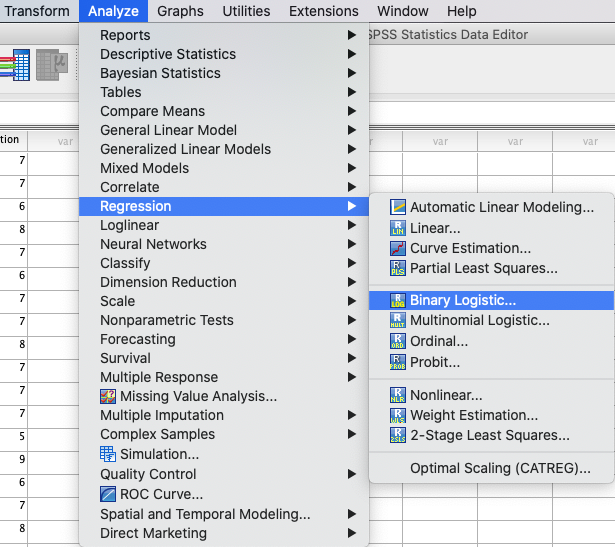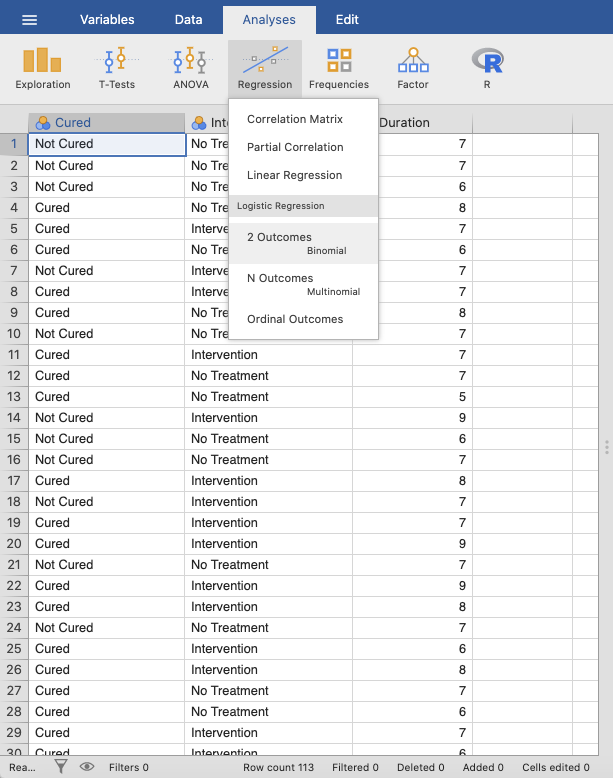In SPSS, move `Cured` to the `Dependent` variable box and `Intervention` to the `Covariates` variable box. In jamovi, move the variable `Cured` to `Dependent Variable` and the variables `Duration` and `Intervention` to `Covariates`.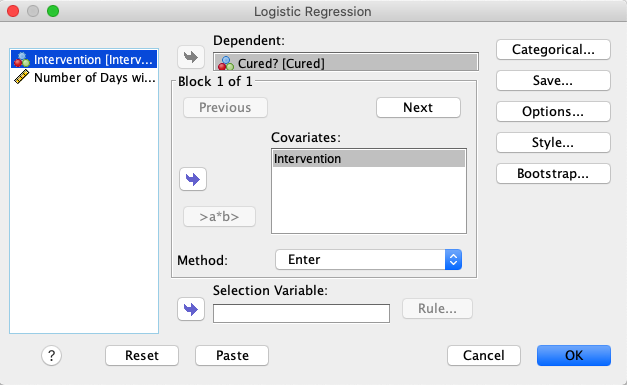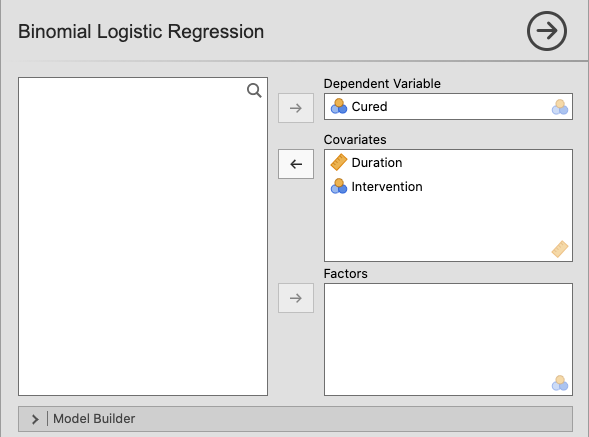Click `Next` and add `Duration` to this new block. Create two new blocks by clicking `+ Add New Block` in the ```Model Builder``` drop-down menu. Add the `Duration` variable to `Block 2`, and add `Duration` and `Intervention` to `Block 3` by marking them both and clicking `Interaction` in the drop-down menu.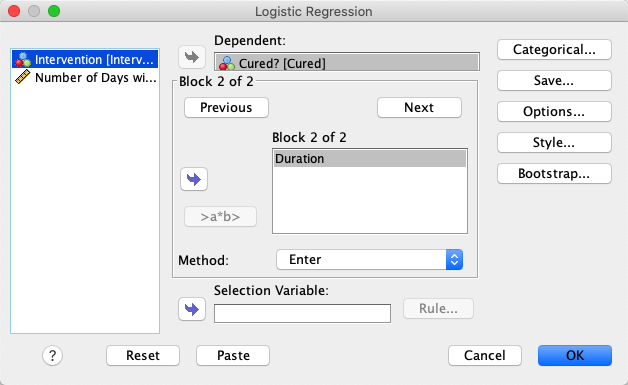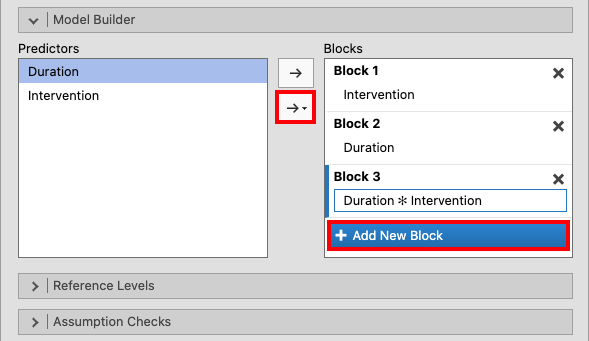Create a third block by clicking `Next` one more time, and create an interaction by marking both `Intervention` and `Duration` and moving them to the block by pressing the arrow.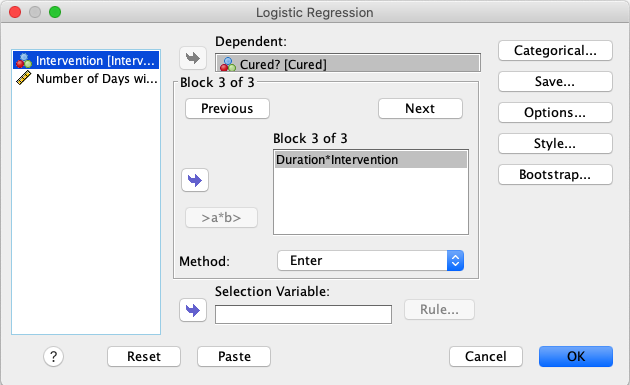Access the `Categorical` window and open the drop-down menu for `Contrast`. Here, choose `Indicator` and then check `First` as the `Reference Category`. Click `Change`. jamovi set the reference category automatically to the first category. If you were to change that, open the drop-down menu `Reference levels`, and change the reference level for each variable to the desired level (e.g., ```Not Cured```).Open the `Options` window and check the following boxes: ```Classification plots```, `Hosmer-Lemeshow goodness-of-fit`, ```Casewise listing of residuals```, `Outliers outside 2 std. dev.`, `Iteration history`, `CI for exp(B)`, `At each step`, and `Include constant in model`. Open the drop-down menu `Prediction` and tick `Classification table`.In the drop-down menu `Model Coefficients`, tick `Odds ratio` and the `Confidence interval` to odds ratio.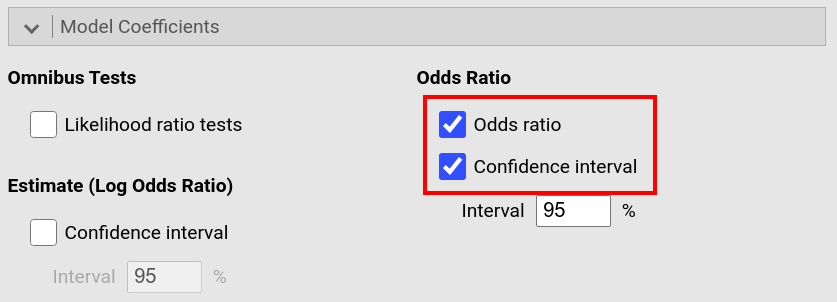In the `Model Fit` window, check the boxes for `Deviance`, `AIC`, `Overall Model Test`, `Cox & Snell's R²` and `Nagelkerke's R²`.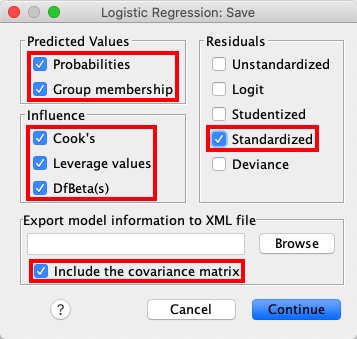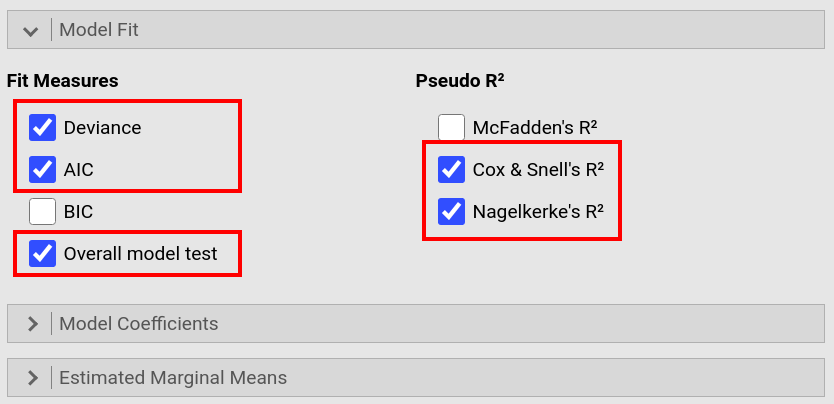Open the `Save` window and check `Probabilities`, `Group membership`, `Cook's`, `Leverage values`, `DfBeta(s)`, `Standardized` (residuals), and `Include the covariance matrix`. jamovi permits you to save some of these values too. To do so, open the drop-down menu `Save` and tick `Predicted values`, `Residuals`, and `Cooks's distance`.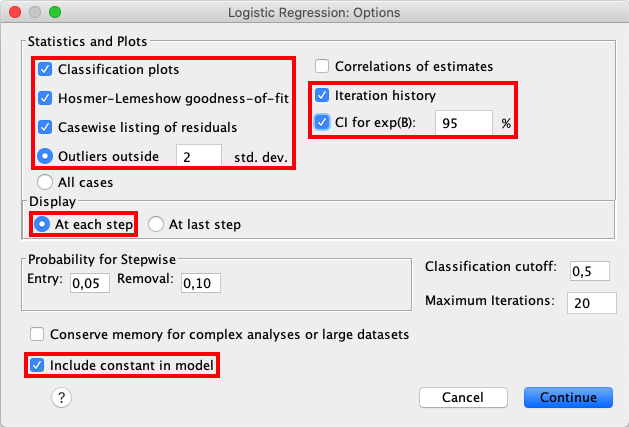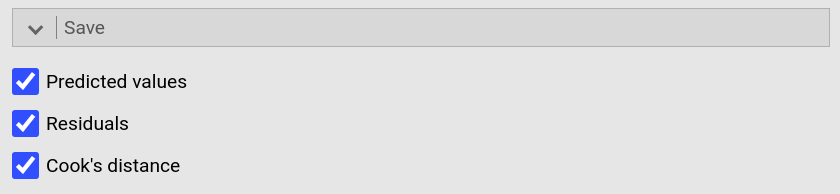If you compare the output from SPSS and jamovi, the results are essentially the same. However, the results from jamovi are shorter and better structured, whereas the SPSS results are much more extensive (likely to the more comprehensive choice of options, according to Field, 2017). jamovi, furthermore, first has an overview over the models and their comparison whereas SPSS provides those model indices within each model.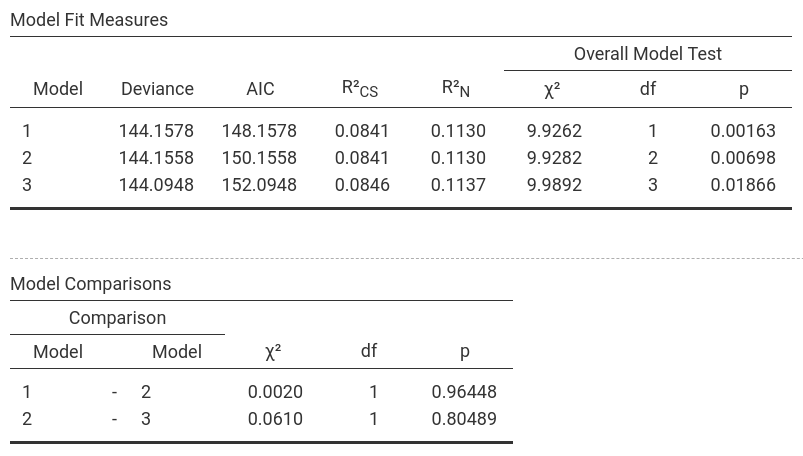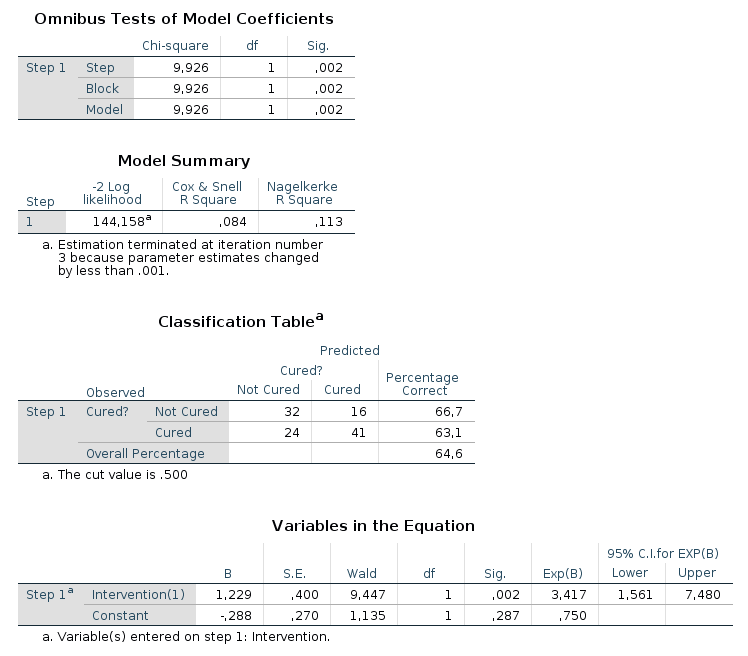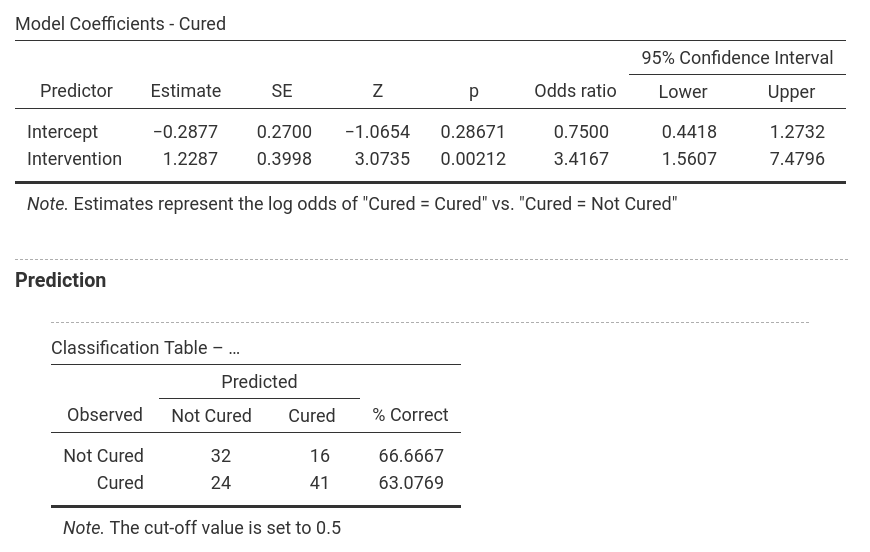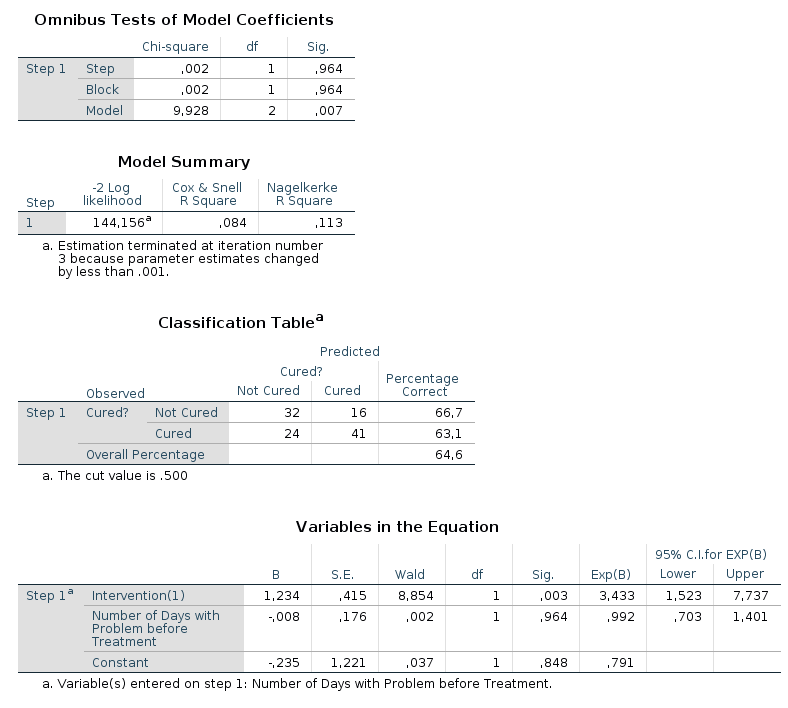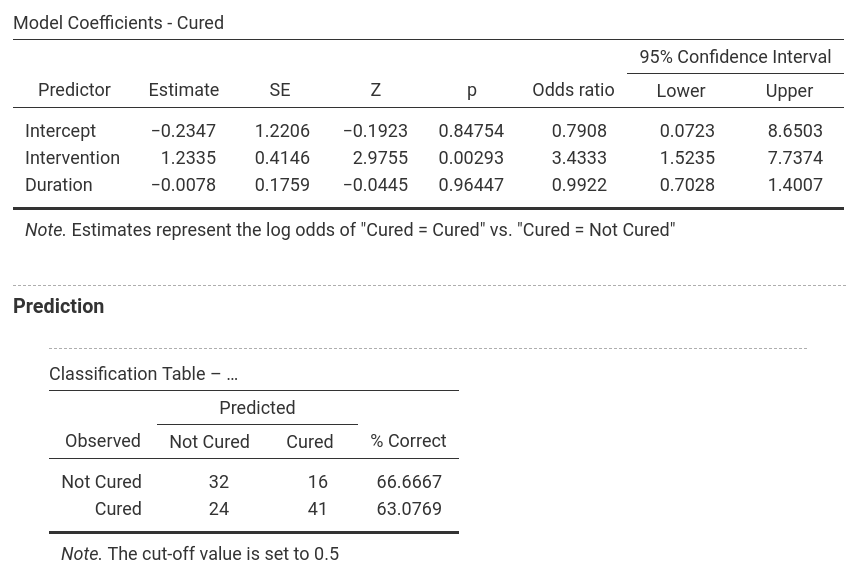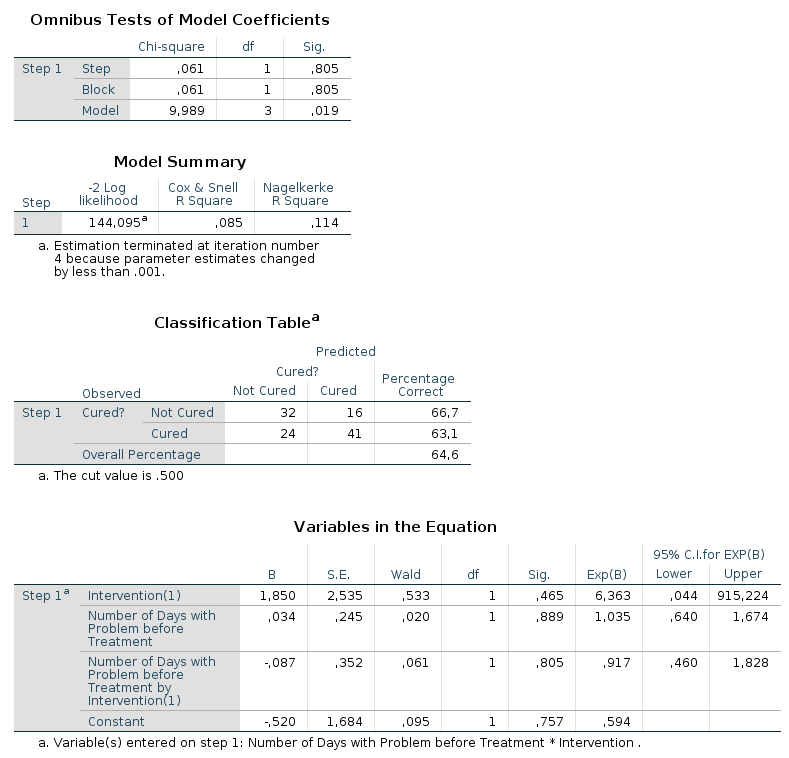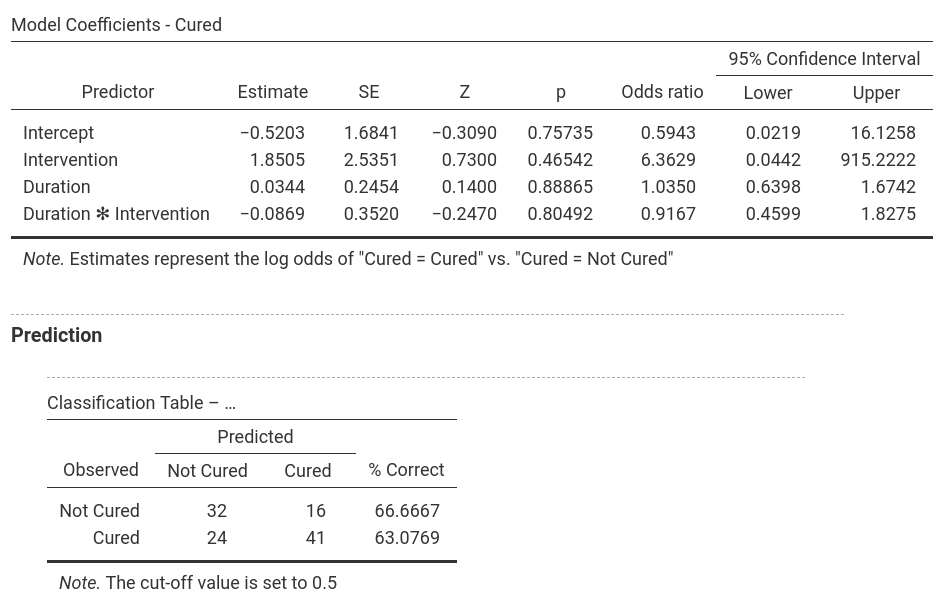In the output from SPSS you can find tables for `Model Summary`, `Variables in the Equation` and `Omnibus Tests of Model Coefficients` for each of the predictors in the model. The `Model Summary` tables shows the -2LL value for the model, as well as Cox & Snell R² and Nagelkerke R². In the `Omnibus Tests of Model Coefficients` tables, χ²-values, degrees of freedom and significance values are found. Lastly, you can find b-values, SE-values, degrees of freedom and significance values in the ```Variables in the Equation``` table. This table also shows the odds ratio. In jamovi, -2LL values, Cox & Snell R² and Nagelkerke R² values for all the predictors are found in the table called `Model Fit Measures`. χ²-values, degrees of freedom and significance levels are found in the ```Model Comparisons``` table. In addition, b-values, SE-values, significance level odds ratio and the confidence interval for it as well as the classification table are shown as separate parts of the output (one for each model). Only one model is shown at a time and the model can be selected by using the drop-down menu next to `Model Specific Results`.
SPSS produces a lot more output tables (some not shown) than jamovi, not the least due to Field (2017) asking for options that are not available in jamovi.
The numerical values for the results are the same.
Model 1: -2LL = 144.158, χ² = 9.926, df = 1, p = 0.002, CS = 0.084, N = 0.113, corr.NC = 66.667, corr.C = 63.077
Model 2: -2LL = 144.156, χ² = 9.928, df = 2, p = 0.007, CS = 0.084, N = 0.113, corr.NC = 66.667, corr.C = 63.077
Model 3: -2LL = 144.095, χ² = 9.989, df = 3, p = 0.019, CS = 0.085, N = 0.114, corr.NC = 66.667, corr.C = 63.077
It becomes clear that `Intervention` is the most decisive predictor whereas `Duration` and the interaction of `Intervention × Duration` don't really lead to better prediction: The number of correctly classified cases doesn't change between the models while Model 1 is the most parsimonuous; furthermore, the Deviance (-2LL) and χ² for Model 2 and 3 are more or less the same as for Model 1 (and since more df's are used in Model 2 and 3, the p-values increase (which is all emphasizing that Model 1 is the best model and should be selected).
If you wish to replicate those analyses using syntax, you can use the commands below (in jamovi, just copy to code below to Rj). Alternatively, you can download the SPSS output files and the jamovi files with the analyses from below the syntax.
```LOGISTIC REGRESSION VARIABLES Cured
/METHOD=ENTER Intervention
/METHOD=ENTER Duration
/METHOD=ENTER Duration * Intervention
/CONTRAST (Intervention)=Indicator(1)
/SAVE=PRED PGROUP COOK LEVER DFBETA ZRESID
/CLASSPLOT
/CASEWISE OUTLIER(2)
/PRINT=GOODFIT ITER(1) CI(95)
/CRITERIA=PIN(0.05) POUT(0.10) ITERATE(20) CUT(0.5).
```
```jmv::logRegBin(
data = data,
dep = Cured,
covs = vars(Duration, Intervention),
blocks = list(list("Intervention"),
list("Duration"),
list(c("Duration", "Intervention"))),
refLevels = list(list(var="Cured", ref="Not Cured")),
pseudoR2 = c("r2mf", "r2cs", "r2n"))
```
SPSS output file containing the analyses jamovi file containing the analyses
References
Field, A. (2017). Discovering statistics using IBM SPSS statistics (5th ed.). SAGE Publications. https://edge.sagepub.com/field5e